# Phase angle of a damped driven harmonic oscillation

• I
• kravky
In summary, In complex equation (4-10), Eulers formula is used to rewrite the equation into two equations. One equation with real part and one equation with imaginary part. Amplitude A is found by multiplying the real part by itself and the imaginary part by itself. For phase angle, the equation is divided into two parts. The first part is found by dividing the real part by the imaginary part. The second part is found by dividing the imaginary part by the real part.f

#### kravky

Hello,
in every book and on every website (e.g. here http://farside.ph.utexas.edu/teaching/315/Waves/node13.html) i found for driven harmonic osciallation the same solution for phase angle:θ=atan(ωb/(k−mω^2)) where ω is driven freq., m is mass, k is spring constant. I agree with it =it follows from solution of diff.eq.of motion

But everywhere i see the same graph for phase angle as a function of ω where phase angle θ goes fromθ=0 when ω=0 thru θ=pi/2 whenω=ω0=resonance, to θ=pi when ω is infinitely large.

How is it possible for phase angle θ be more than pi/2 if θ=atan(ωb/(k−mω^2))
Is it not arctan and is it not limited from -pi/2 to pi/2 ?

here u can see the classical picture (second one - right one). phase angle there is phi instead of theta (just another symbol but same meaning) and u can see it in dimensionless values. left picture is amplitude as a function of ω. IT makes sense. But i don't understand the second one.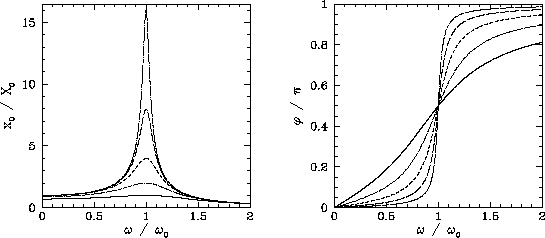Also here: http://lampx.tugraz.at/~hadley/physikm/apps/resonance.en.php. This formula does not work.
it has to be sth else that pure arctang. it is ok for x greater than 0 (arctg(x)) but for x less then 0 this forumla doesn't work. it has to be like θ=pi-atan(ωb/(k−mω^2))

I think it cannot be pure arctan. it has to be theta=pi - atan(ωb/(k−mω^2)) for argument (ωb/(k−mω^2)) less than zero. But i don't know why.
What is worng with that? Simply: arctan has range from -pi/2 to pi/2. So i am really confused how it can be pi according to books and websites.

*phase angle=angle by which the driving force leads the displacement of the system

#### Attachments

For frequencies greater than ## \omega_o ##, the amplitude is negative, which can be converted to positive by making the minus sign into a ## e^{i \pi} ## in the solution of the differential equation in the complex form. ## \\ ## If you really want to see what is going on with the phase angle, begin with ## m \ddot{x}+b \dot{x}=kx=F_o e^{+i \omega t} ##, and let the steady state solution be of the form ## x=Ae^{+i \omega t} ##, and work through the algebra by taking derivatives, and solving for ## A ## as a complex number with an amplitude and a phase. Finally take the real part of it to get ##x ## in the form ## x_{real}=A_o cos(\omega t-\phi) ## where ## A_o ## is a real and positive amplitude. (Again, to convert ## A ## into a positive amplitude if it has a minus sign out front in the real part, change that minus sign to a factor of ## e^{i \pi} ##, (factoring a "-1"=##e^{i \pi} ## out of the complete expression for ## A ##). When it is in the form ## x=A_o e^{i (\omega t-\phi)} ##, take the real part to give ## x_{real}=A_o cos(\omega t-\phi) ##). ## \\ ## For some additional details: ## A=\frac{F_o}{-m \omega^2+k+i b \omega}=\frac{F_o(-m \omega^2+k- i b \omega)}{(m \omega^2+k)^2+b^2 \omega^2} ##. The relevant phase information, including any minus signs is all in the term in the numerator ## -m \omega^2+k-i b \omega ##. When ## m \omega^2 >k ##,(for frequencies above resonance), the result is ## e^{i \pi} (m \omega^2-k +ib \omega) ## so that ## x=A_o e^{i( \pi+\delta)} e^{i \omega t} ##, for some ## \delta ## where ## 0<\delta < \frac{\pi}{2} ##. ## \\ ## Taking the real part of ## x ##: ## x_{real}=A_o cos(\omega t+(\pi+\delta))=A_o cos(\omega t-(\pi-\delta)) ##, where we have used ## cos(\theta+\pi)=cos(\theta-\pi) ##. The expression has the form ## x_{real}=A_o cos(\omega t-\phi) ## where ## \phi=\pi-\delta ##. The angle ## \delta ## goes to zero for very high frequencies.

Last edited:
•kravky
Well, i did it pretty much same way but i didnt get negative Amplitude. I think i did algebra correctly but i got positive amplitude.
I followed this: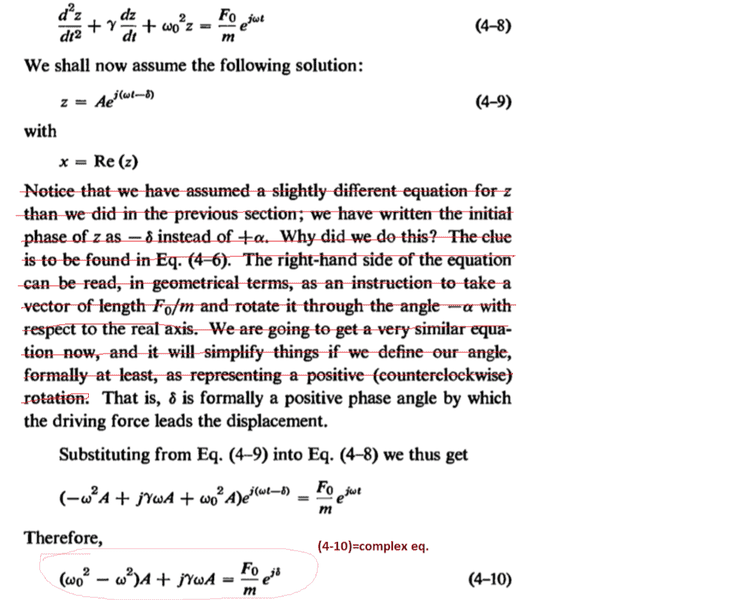-------------------------------------------------------------------------------------------------------------------------------------------------------------------------------------------------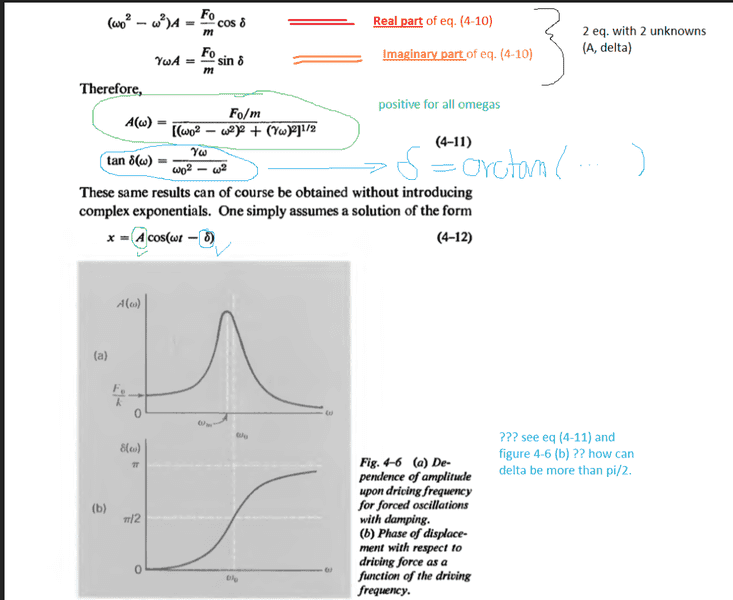---------------------------------------------------------------------------------------------------------------------------------------------------------------------------------------------------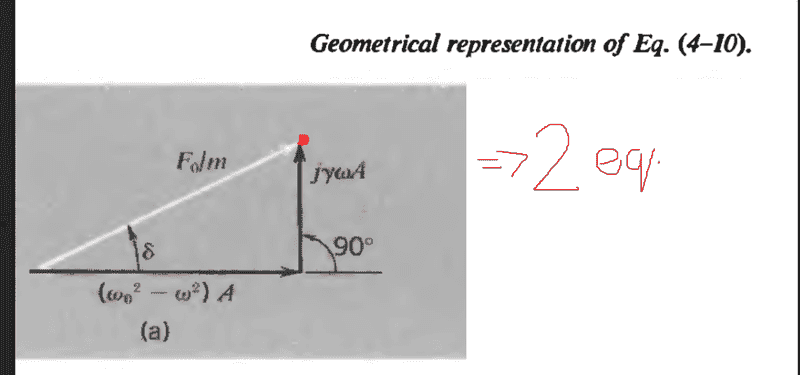** in complex eq.(4-10) i used Eulers formula for rewriting it:and then got 2 equations from eq. (4-10). For real and for imaginary part.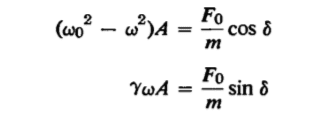So 2 eq. with two unknows: Amplitude A and phase angle δ. Its very easy to solve:

for Amplitude A I just squared them both and then add them together. and A popped out.

For phase angle delta i just devided these 2 eq.

----
Here is my solving: Real part of eq. (4-10) is marked as (6) and Imaginary part as eq. number (7)

-I use defferent symbols! = different notation. i use 2δ instead of γ (i don't use gama)
and i use φ instead of δ as phase angle!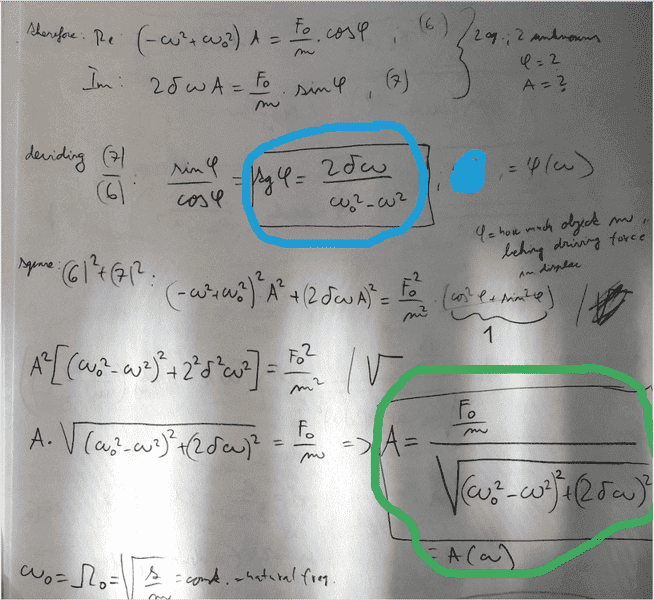and u see i get positive amplitude for all driving freq. omegas and therefore probably worng eq. for phase angle.

#### Attachments

The equation that you get from setting the imaginary parts equal ## \gamma \omega A=\frac{F_o}{m} \sin{\delta} ##, tells you ## 0<\delta< \pi ##, if you go under the assumption that ## A>0 ##, which it must be from your formula for ## A ##. With this equation, it no longer allows you the freedom of taking the domain of ## \delta(\omega) ## in the subsequent ## \tan{\delta(\omega)}=\frac{\gamma \omega}{\omega_o^2-\omega^2} ## formula as being from ## -\frac{\pi}{2} ## to ## +\frac{\pi}{2} ##.

Last edited:
Is my solution incorrect? Have i made wrong assumptions? Because this solution is also in book Vibration and Waves by A.P. French.
If i plot his=my solution for phase angle , the graph looks nonphysically discountious. Look: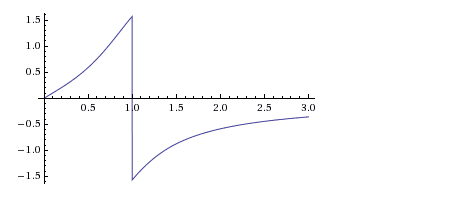(plotted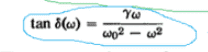in dimensionless numbers)

However he does not present this incorrect grpah, he present the correct continuous graph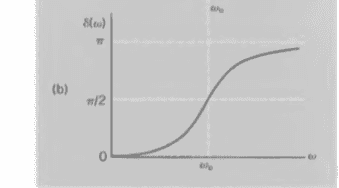, although it does not correspond with his equation for phase angle. Its strange. He probably knows how graph of phase angle looks like, but his solution is certainly not OK.

Sir, could You please explain how Did u got this term for amplitude?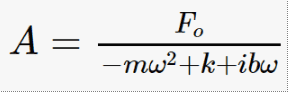because your solution that u posted at 1:37 AM seems to be correct.

Thanks a lot for your time

#### Attachments

Using ## x=Ae^{i \omega t} ##, and using the differential equation of post 2 above, ## \ddot x=\frac{d^2 x}{dt^2}=- \omega ^2 Ae^{i \omega t} ##. Similarly, ## \dot{x}=\frac{dx}{dt}=+i \omega A e^{i \omega t} ##. Here ## A ## is a complex constant that includes the phase. ##A## can also be frequency dependent. The ## e^{i \omega t} ## factors out of both sides of the equation and cancels. On the left side, ## A ## also factors out, making it ## A(-m \omega^2+i b \omega+k)=F_o ##. ## \\ ##You should note, the differential equation comes from ## F=ma ##, so that ## m \ddot{x}=F_o e^{i \omega t}-b \dot{x}-kx ##. The force of a spring is ## F_s=-kx ## and the velocity dependent damping force is ## F_d=-b \dot{x} ##. Thereby, the differential equation ## m \ddot{x}+b \dot{x}+kx=F_o e^{i \omega t} ## makes perfect sense. The applied force is ## F_oe^{i \omega t} ##.

And a follow-on: I have seen that resonant type graph with the discontinuity you show in the amplitude in books describing magnetic resonance, (a book by C.P. Slichter), and the behavior of the susceptibility function ## \chi(\omega) ##, where ## m(\omega)=\chi(\omega) h(\omega) ##, where ## h(\omega) ## is an applied r-f magnetic field at right angles to a very strong and static ## H_o ## magnetic field. (Edit: I subsequently looked up the graphs in the book by C.P. Slichter, and he has graphs of the real part of ## \chi(\omega ) ## vs. frequency (## \omega ##), and also of the imaginary part of ## \chi(\omega) ## as a function of frequency. My original statement is in error on this item=his graphs are correct and do not have any discontinuity). ## \\ ## I have also seen an experimental laboratory set-up with a sinusoidal driving force of a mass on a spring system on a nearly frictionless rail, and the system does acquire a very large amplitude at and near resonance. In this experiment, there was a sinusoidal driving motion on the right end connection of the spring at the end of the rail, (the left side also had a spring connected to it, so that the mass sits on the rail between two springs), which thereby was a sinusoidal driving force, and the frequency of the driving force was adjustable. ## \\ ## What you are asking is an interesting question: Is there an experimentally observed discontinuity in these systems? For frequencies just below resonance, the amplitude is positive, at the phase delay is just less than ## + \frac{\pi}{2} ##, while just above resonance, the amplitude is negative, but if this is corrected and made positive by the ## e^{i \pi} ## in my derivation, it appears the phase lag angle is just slightly greater than ## + \frac{\pi}{2} ##. If I am interpreting my calculations correctly, no physical discontinuity is observed as you pass through the resonance.

Last edited:
•kravky
I understand where does this diff. eq. comes from. I just don't understand correct solution method. Maybe i should study more complex numbers.

i overlooked that u took x=Aeiωt. I took x=Aei(ωt-φ) so i got different answer.
i considered also phase angle in trial function x=Aei(ωt-φ) for steady state solution of differential equation.

•I understand where does this diff. eq. comes from. I just don't understand correct solution method. Maybe i should study more complex numbers.

i overlooked that u took x=Aeiωt. I took x=Aei(ωt-φ) so i got different answer.
i considered also phase angle in trial function x=Aei(ωt-φ) for steady state solution of differential equation.
The derivative of ## y=e^{\alpha t} ## is ## \frac{dy}{dt}=\alpha e^{\alpha t} ##. That works even in the case where ## \alpha=i \omega ##, so that ## \alpha ## is a complex number. ## \\ ## e.g. If ## y=A e^{i \omega t} ## , then ## \frac{d y}{dt}= i \omega A e^{i \omega t} ##. ## \\ ## And please see my post 7 above.

For frequencies just below resonance, the amplitude is positive, at the phase delay is just less than ## + \frac{\pi}{2} ##, while just above resonance, the amplitude is negative, but if this is corrected and made positive by the ## e^{i \pi} ## in my derivation, it appears the phase lag angle is just slightly greater than ## + \frac{\pi}{2} ##. If I am interpreting my calculations correctly, no physical discontinuity is observed as you pass through the resonance.

Well here is MIT professor talking about this problem. At 18min. he is talking about phase angle. However it is same as my solution...
In comment section u can see one person asks same question as me (

Huihuang LaiPred 1 year ago
Sir, at 18:28 how can delta be equal to pi? Isn't delta an arctan, which has range from -pi/2 to pi/2? pi seems out of range.)
Unfortunatelly he doesn't get explanation.

regarding continuity of the system: The Reality is = No discontinuity. How on Earth could be phase angle not only discontinuous but also negative. It would physically mean the mass-spring system is oscillating BEFORE driving force.

But derviations according to Vibration and Waves by A.P. French give discontiunity as i showed u on the grpahs. At least his equations give discontinuity. His graphs present correct dependence of phase angle on driving freq. = continuous.

Your derivation is simply different and correct.

See my post 4. I think that may help answer your question here.

•kravky
See my post 4. I think that may help answer your question here.
Yes, i kindly appreciate your help sir. now i understand it much better. I just don't know why books don't use ,,your,, derivation.

•Hello,
in every book and on every website (e.g. here http://farside.ph.utexas.edu/teaching/315/Waves/node13.html) i found for driven harmonic osciallation the same solution for phase angle:θ=atan(ωb/(k−mω^2)) where ω is driven freq., m is mass, k is spring constant. I agree with it =it follows from solution of diff.eq.of motion

But everywhere i see the same graph for phase angle as a function of ω where phase angle θ goes fromθ=0 when ω=0 thru θ=pi/2 whenω=ω0=resonance, to θ=pi when ω is infinitely large.

How is it possible for phase angle θ be more than pi/2 if θ=atan(ωb/(k−mω^2))
Is it not arctan and is it not limited from -pi/2 to pi/2 ?

here u can see the classical picture (second one - right one). phase angle there is phi instead of theta (just another symbol but same meaning) and u can see it in dimensionless values. left picture is amplitude as a function of ω. IT makes sense. But i don't understand the second one.

View attachment 230453

Also here: http://lampx.tugraz.at/~hadley/physikm/apps/resonance.en.php. This formula does not work.
it has to be sth else that pure arctang. it is ok for x greater than 0 (arctg(x)) but for x less then 0 this forumla doesn't work. it has to be like θ=pi-atan(ωb/(k−mω^2))

I think it cannot be pure arctan. it has to be theta=pi - atan(ωb/(k−mω^2)) for argument (ωb/(k−mω^2)) less than zero. But i don't know why.
What is worng with that? Simply: arctan has range from -pi/2 to pi/2. So i am really confused how it can be pi according to books and websites.

*phase angle=angle by which the driving force leads the displacement of the system
Well, you are a victim of one of the few really mortal sins of physics didactics. That's the claim that you can easily figure out a polar angle using the formula with the tangens. To get it right you need sin and cos and a definition over which semi-open interval of length ##2 \pi## the angle should run (which is of course completely arbitrary). In the context of (relative) phase shifts the most intuitive choice is the interval ##(-\pi,\pi]##.

If $$(r,\varphi)$$ are the standard polar coordinates in a plane, related to the Cartesian coordinates via
$$(x,y)=r (cos \varphi,\sin \varphi),$$
you get the right angle ##\varphi## in this interval by first calculating
$$r=\sqrt{x^2+y^2}.$$
Then the angle is given by the ##x## value alone only up to its sign, but it's easy to determine the sign from the ##y## value, since obviously it's just given by the sign of the ##y## value (it's easist to see with a little drawing). Thus you get
$$\varphi=\arccos \left (\frac{x}{r} \right) \text{sign} y.$$
There's only an ambiguity for ##y=0##. Then you have ##\frac{x}/r=x/|x| =\text{sign} x##. For ##x=r>0## you uniquely get ##\varphi=0##, for ##x=-r<0## by definition we choose ##+\pi##.

Writing the driving force as
$$F=F_0 \cos(\omega t),$$
and the stationary solution as
$$x=x_0 \cos(\omega t-\varphi_0),$$
you always get ##\varphi \geq 0## (i.e., the phase of the motion always lags behind the driving force, which is also pretty intuitive). The correct formula is (in the notation of the eom. in the link in the OP)
$$\varphi_0=+\arccos \left (\frac{\omega_0^2-\omega^2}{\sqrt{(\omega_0^2-\omega^2)^2+\omega^2 \nu^2}} \right),$$
which shows that you get ##\varphi_0=\pi/2## for ##\omega=\omega_0##. For ##\omega \rightarrow 0## the phase shift goes to 0 and for ##\omega \rightarrow \infty## it goes to ##\pi##, as shown in the plots cited in this thread.

•